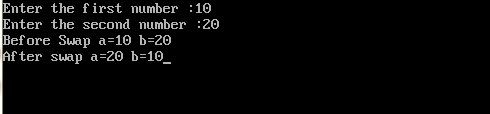UncleCoder.com

UncleCoder.com

Free programming examples and instructions

# C Program to swap two numbers using Pointers

## C Program for how to swap two numbers using Pointers

by Krishna

Posted on 27 Jun 2018 Category: C Views: 1305

C Program to swap two numbers using Pointers

In this sectionwe will write a C program to swap two numbers using Pointers.

``````#include <stdio.h>

// function to swap the two numbers
void swap(int *x,int *y)
{
int t;
t   = *x;
*x   = *y;
*y   =  t;
}

int main()
{
int a,b;

printf("Enter the first number: ");
scanf("%d",&a);
printf("Enter the second number: ");
scanf("%d",&b);

//displaying numbers before swapping
printf("Before Swap: a = %d, b= %d\n",a,b);

//calling the user defined function swap()
swap(&a,&b);

//displaying numbers after swapping
printf("After  Swap: a= %d, b=%d\n",a,b);

return 0;
}``````

OUTPUT